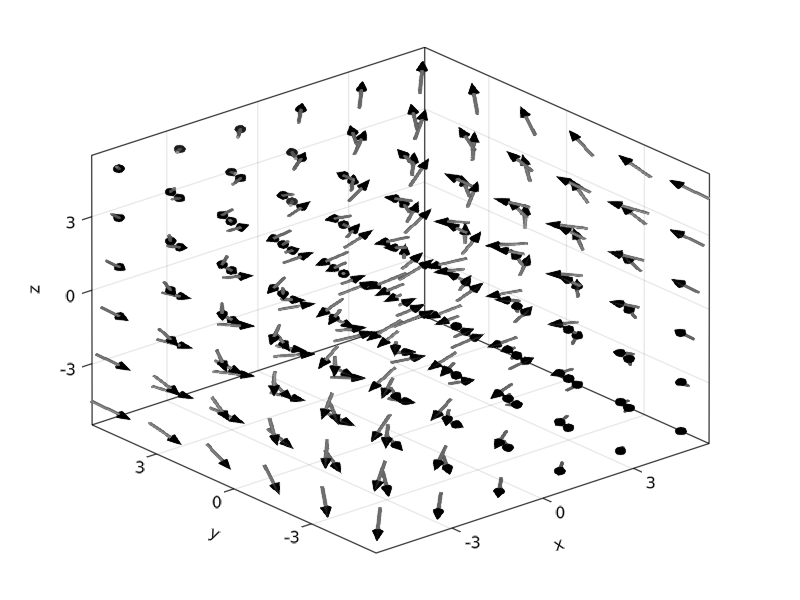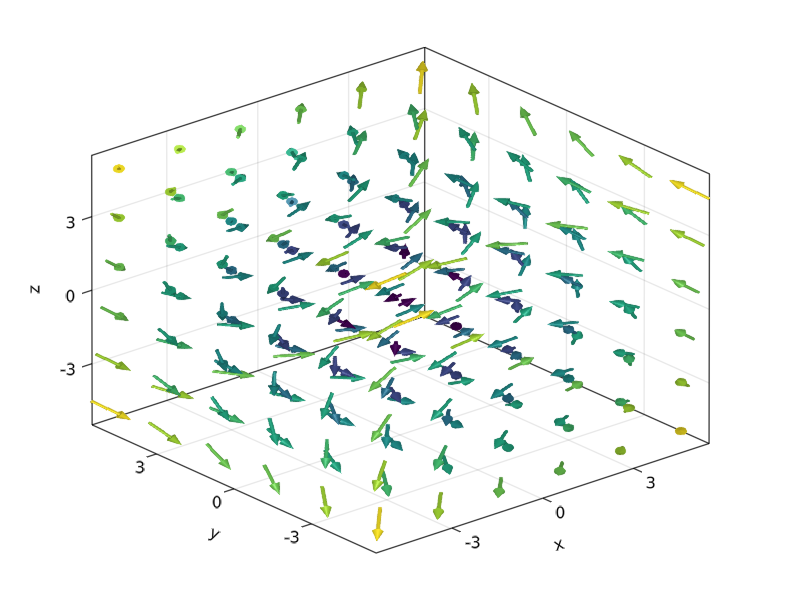# arrows

Makie.arrowsFunction
arrows(points, directions; kwargs...)
arrows(x, y, u, v)
arrows(x::AbstractVector, y::AbstractVector, u::AbstractMatrix, v::AbstractMatrix)
arrows(x, y, z, u, v, w)

Plots arrows at the specified points with the specified components. u and v are interpreted as vector components (u being the x and v being the y), and the vectors are plotted with the tails at x, y.

If x, y, u, v are <: AbstractVector, then each 'row' is plotted as a single vector.

If u, v are <: AbstractMatrix, then x and y are interpreted as specifications for a grid, and u, v are plotted as arrows along the grid.

arrows can also work in three dimensions.

Attributes

Available attributes and their defaults for Combined{Makie.arrows, T} where T are:

  align           :origin
ambient         Float32[0.55, 0.55, 0.55]
arrowcolor      MakieCore.Automatic()
arrowhead       MakieCore.Automatic()
arrowsize       MakieCore.Automatic()
arrowtail       MakieCore.Automatic()
color           :black
colormap        :viridis
diffuse         Float32[0.4, 0.4, 0.4]
inspectable     true
lengthscale     1.0f0
lightposition   :eyeposition
linecolor       MakieCore.Automatic()
linestyle       "nothing"
linewidth       MakieCore.Automatic()
markerspace     Pixel
nan_color       RGBA{Float32}(0.0f0,0.0f0,0.0f0,0.0f0)
normalize       false
overdraw        false
quality         32
shininess       32.0f0
specular        Float32[0.2, 0.2, 0.2]
ssao            false
transparency    false
visible         true
source

### Attributes

• arrowhead = automatic: Defines the marker (2D) or mesh (3D) that is used as the arrow head. The default for is '▲' in 2D and a cone mesh in 3D. For the latter the mesh should start at Point3f0(0) and point in positive z-direction.
• arrowtail = automatic: Defines the mesh used to draw the arrow tail in 3D. It should start at Point3f0(0) and extend in negative z-direction. The default is a cylinder. This has no effect on the 2D plot.
• quality = 32: Defines the number of angle subdivisions used when generating the arrow head and tail meshes. Consider lowering this if you have performance issues. Only applies to 3D plots.
• linecolor = :black: Sets the color used for the arrow tail which is represented by a line in 2D.
• arrowcolor = linecolor: Sets the color of the arrow head.
• arrowsize = automatic: Scales the size of the arrow head. This defaults to 0.3 in the 2D case and Vec3f0(0.2, 0.2, 0.3) in the 3D case. For the latter the first two components scale the radius (in x/y direction) and the last scales the length of the cone. If the arrowsize is set to 1, the cone will have a diameter and length of 1.
• linewidth = automatic: Scales the width/diameter of the arrow tail. Defaults to 1 for 2D and 0.05 for the 3D case.
• lengthscale = 1f0: Scales the length of the arrow tail.
• linestyle = nothing: Sets the linestyle used in 2D. Does not apply to 3D plots.
• normalize = false: By default the lengths of the directions given to arrows are used to scale the length of the arrow tails. If this attribute is set to true the directions are normalized, skipping this scaling.
• align = :origin: Sets how arrows are positioned. By default arrows start at the given positions and extend along the given directions. If this attribute is set to :head, :lineend, :tailend, :headstart or :center the given positions will be between the head and tail of each arrow instead.

### Examples

using CairoMakie

f = Figure(resolution = (800, 800))
Axis(f[1, 1], backgroundcolor = "black")

xs = LinRange(0, 2pi, 20)
ys = LinRange(0, 3pi, 20)
us = [sin(x) * cos(y) for x in xs, y in ys]
vs = [-cos(x) * sin(y) for x in xs, y in ys]
strength = vec(sqrt.(us .^ 2 .+ vs .^ 2))

arrows!(xs, ys, us, vs, arrowsize = 10, lengthscale = 0.3,
arrowcolor = strength, linecolor = strength)

fusing GLMakie

ps = [Point3f0(x, y, z) for x in -5:2:5 for y in -5:2:5 for z in -5:2:5]
ns = map(p -> 0.1 * Vec3f0(p, p, p), ps)
arrows(
ps, ns, fxaa=true, # turn on anti-aliasing
linecolor = :gray, arrowcolor = :black,
linewidth = 0.1, arrowsize = Vec3f0(0.3, 0.3, 0.4),
align = :center, axis=(type=Axis3,)
)using LinearAlgebra
lengths = norm.(ns)
arrows(
ps, ns, fxaa=true, # turn on anti-aliasing
color=lengths,
linewidth = 0.1, arrowsize = Vec3f0(0.3, 0.3, 0.4),
align = :center, axis=(type=Axis3,)
)